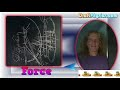\$2,000 Challenge Website 2.0 | DebatePhysics.comThe Naked Science Forum Transcript

 RE: Preston Becker ...Veritasium Electricity explained ...part 2 [5/14/22]

What Is Gravity? ...The sensible answerWhat Is A Photon? ...The sensible answer

Single slit facts

1. The single slit math models two vectors with Point sources at the surfaces. -- That is, the math models two diffracting "waves" with their centers at the surfaces.

2. The math predicts/models a result on a curved surface of radius L (target distance).

3. The number of possible nodes/locations of constructive or destructive interference is defined by how many wavelengths of the light fit in the distance between the surfaces. ... Dividing the wavelength into the distance between the surfaces will tell you exactly how many on/off nodes will be projected onto the curved surface.

4. The "path length difference" at 90° is the total distance between the surfaces and dictates what the node at 0° will be.

5. The equidistant vectors pointing to the center do not create real nodes as they only span a tiny distance (the distance between two surfaces) at the Target. Real vectors move in the same left/right direction.

7. The double slit experiment is just two single slit experiment superimposed on top of each other. Not to single slits of the same dimensions, but rather a composite product of a single slit with the dimensions of the center impediment and a single slit with the dimensions of the outside surfaces. ... The double slit is combining two, two Source, experiments... Where the four sources are in phase there is construction of a photon... When all four sources are not in phase no photon is recorded.